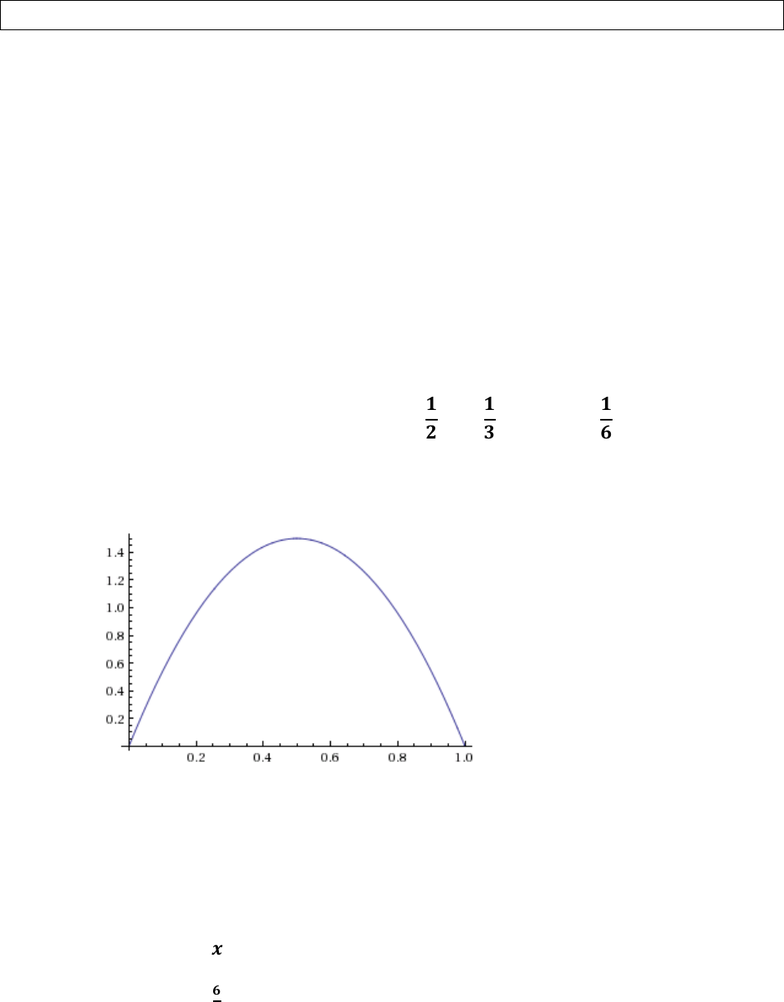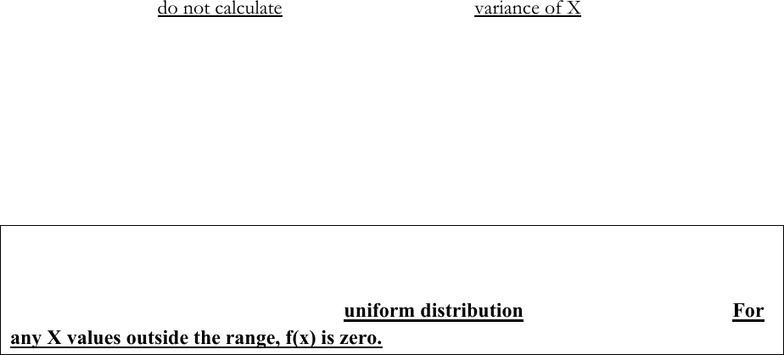Get 2 days of unlimited access
Class Notes (1,000,000)
CA (600,000)
York (40,000)
MGMT (700)
Lecture 11

# MGMT 4800 Lecture Notes - Lecture 11: Probability Distribution, Standard DeviationExam

Department
Management
Course Code
MGMT 4800
Professor
Joliuna
Lecture
11

This preview shows pages 1-2. to view the full 7 pages of the document.STAT 1430 REC 10A (SOLUTIONS) SEC 4.3
CONTINUOUS R.V.
1. A fast food manager wants to be prepared to have enough of COIN change (pennies,
nickels, dimes, and quarters) at the drive through window ready to give to customers. Let X
be the amount of COIN change that any customer is given back when they pay for their
food at the drive up window (in decimal form.) Note: The amount of coin change would be
anywhere from 0 cents to 99 cents = .99 dollars. Suppose f(x) is below.
f(x) = 6x(1-x) where 0 ≤ x < 1.00 (in decimal form)
a) Find the value of k that makes this a legitimate pdf.
All pdfs integrate to 1. Therefore we need to solve the following equation for k:
 

The integral equals
 

Therefore, .
b) Graph f(x)
c) Show that f(x) is a legitimate pdf for X.
i. f(x)≥0 for all x from 0 to 1
ii. 


d) Find the mean amount of change a customer receives back when paying for their order.
 






Only pages 1-2 are available for preview. Some parts have been intentionally blurred.STAT 1430 REC 10A (SOLUTIONS) SEC 4.3
CONTINUOUS R.V.
e) Using your graph from part (a) and the fact that this distribution is symmetric, what is
the median of this distribution?
The median is 0.5. Much like when we had a uniform distribution, the median
was simply the midpoint of possible values of X. This is the case when we have
symmetric distributions.
f) We could have used integration to find the median, but why didn’t we need to in this
case? How is this different from #1 above?
We didn’t need to use integration because the distribution is symmetric (like the
uniform). The pdf in #1 is not symmetric however. Therefore the midpoint of
possible values won’t be the median, because there will be more area on one side
of the midpoint than the other.
g) Set up but do not calculate how we would find the variance of X.


h) Then show how we take that result to find the standard deviation (use proper units.)
To get , we take the square root of
. The units for is dollars in coin
change.
UNIFORM DISTRIBUTION
Suppose X is a continuous random variable whose pdf is a flat line between two values a
and b. In this case we say that X has a uniform distribution over the interval [a, b]. For
any X values outside the range, f(x) is zero.
NOTE: ALWAYS DRAW PICTURES WHEN SOLVING PROBLEMS LIKE THE
ONES BELOW. IT WILL HELP YOU AND WE’LL BE EXPECTING TO SEE THEM.
For the next 5 problems: Suppose a manager of a phone helpline wants to limit the time a
customer has to wait on hold to less than 10 minutes. Assuming this actually happens, let X be
the amount of time a customer is on hold. Assume X has a uniform distribution over the interval
[0, 10].
2. Graph f(x).
###### You're Reading a Preview

Unlock to view full version